# ICSE Worksheet for Chapter - 12 Mensuration Class 8

## Find ICSE Worksheet for chapter-12 Mensuration class 8

CLASS-8

BOARD: ICSE

Mathematic Worksheet - 12

TOPIC: Mensuration

For otherICSE Worksheet for class 8 Mathematic check out main page of Physics Wallah.

#### SUMMARY

Triangle:

Perimeter = a + b + c

Area = 1/2×Base×Height=1/2 ah

Heron’s formula:

Area = √[(s(s-a)(s-b)(s-c)]

Where, s = semi perimeter = (a + b + c)/2

Area of equilateral triangle = √3/4 (side)2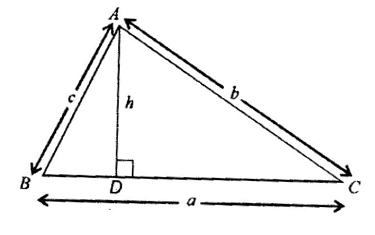Rectangle:

Perimeter = 2(l +b)

Area = l × b

Length of diagonal = √(l2+b2 )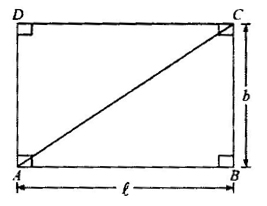Square:

Perimeter = 4a

Area = a2

Length of diagonal = a√2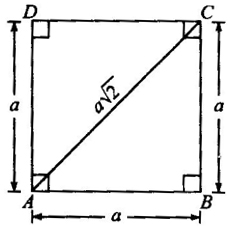Parallelogram:

Perimeter = 2(a+b)

Area = ah1 = bh2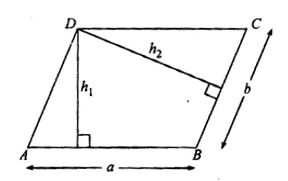Rhombus:

Perimeter = 4a = 2√(d12+d22 )

Area = 1/2 d1 d2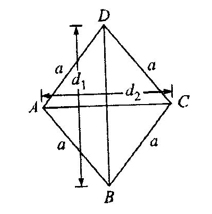Let AC = d

Area = 1/2 d(h1 h2 )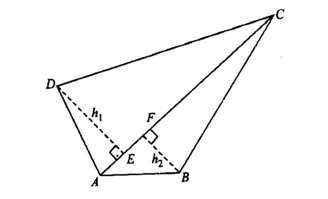Trapezium:

Area = 1/2 h(a+b)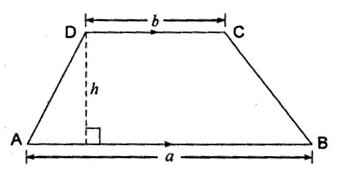Circle:

Area of circle (A) = πr2

Circumference (C) = 2πr

Diameter (D) = 2r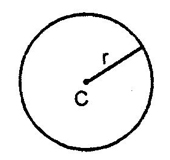Cuboids:

Total Surface Area (T.S.A.): The area of surface from which cuboids is formed. There are six faces (rectangular), eight vertices and twelve edges in a cuboids.

Total Surface Area (T.S.A.) = 2[l×b+b×h+h×l]

Lateral Surface Area(L.S.A.) = 2h[l+b]

Volume of cuboids = l×b×h

Length if diagonal = √(l2+b2+h2 )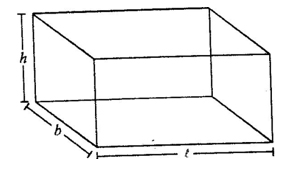Cylinder:

i. C.A.S. of cylinder = (2πr)×h=2πrh

ii. Total Surface Area (T.S.A.):

T.A.S. = C.A.S. + area of circular top & bottom

= 2πr(h+r)

iii. Volume of cylinder = Area of base × height

=πr2 h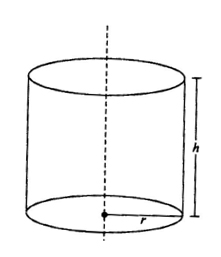Sphere:

T.S.A. = S.A. = 4πr2

Volume = 4/3 πr3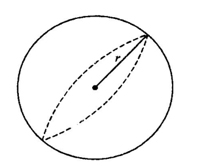1. The length and breadth of a rectangular plot are 900 m and 700 m respectively. If three rounds of fence is fixed around the field at the cost of Rs 8 per meter, the total amount spent is:

(A)Rs 768

(B) Rs 7680

(C) Rs 76800

(D) Rs 768000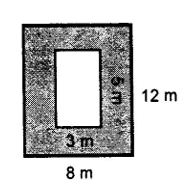2. The area of the shaded region is:

(A) 96 sq. m

(B) 15 sq. m

(C) 81sq. m

(D) 111 sq. m

3. The difference between the length and breadth of a rectangle is 23 m. if perimeter is 206 m, then the area is:

(A) 1520sq. m

(B) 2520 sq. m

(C) 2420 sq. m

(D) None of these

4. The perimeter of a trapezium is 52 cm and its non-parallel sides are each equal to 10 cm and its altitude is 8 cm. its area is:

(A) 128 cm2

(B) 112 cm2

(C) 118 cm2

(D) 124cm2

5. If the ratio of circumference of two circles is 4 : 9, the ratio of their area is:

(A) 9:4

(B) 16:81

(C) 4:9

(D) 2:3

6. A wire bent in the form of a circle of radius 42 cm is cut and again bent in form of a square. The ratio of the region enclosed by the circle and the square in the two cases is given by:

(A) 11:22

(B) 21:33

(C) 22:33

(D) 14:11

7. A horse is tethered to a corner of a field which is in the shape of an equilateral triangle, if the length of the rope to which it is tied is 7 m, so the area of the field over which it grazes is:

(A) 49 cm2

(B) 22 2/3 cm2

(C)25 2/3 cm2

(D) None of these

8. A man walked 20 m to cross a rectangular field diagonally. If the length of the field is 16 m, the breadth of the field is:

(A) 4 m

(B) 16 m

(C) 12 m

(D) Cannot be determined

9. In the given figure, OPQR is a rhombus, three of whose vertices lie on a circle with centre O. if the area of rhombus is32√3 cm2. The radius of circle is:

(A) 8

(B) 9

(C) 10

(D) 11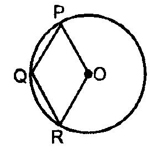10. A wire in the shape of an equilateral triangle encloses an area of S sq. cm. if the same wire is bent to form of a circle. The area of the circle will be:

(A) (πS2)/9

(B) (3S2)/π

(C) 3S/π

(D) (3√3 S)/π

11. Three cubes whose edges are 3 cm, 4 cm, and 5 cm respectively are melted to form a single cube. Find the surface area of the new cube.

(A)210 cm2

(B) 213 cm2

(C) 224 cm2

(D) 216 cm2

12. A hemispherical bowl of internal diameter 36 cm is full of some liquid. This liquid is to be filled in cylindrical bottles of radius 3 cm and height 6 cm, then no of bottles needed to empty the bowl.

(A) 36

(B) 72

(C) 18

(D) 144

13. The total surface area of a cube is numerically equal to the surface of a sphere then the ratio of their volume is:

(A) π/6

(B) √(π/6)

(C) π/216

(D) √(6/π)

14. In the adjoining figure, the radius of the inner circle, if other circles are of radii 1m, is: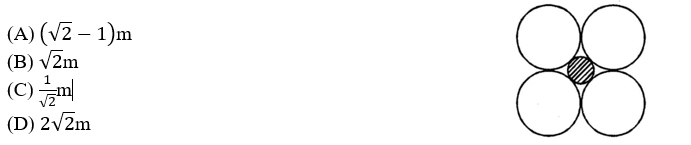15. A square, a rectangle and a right angled isosceles triangle have the same perimeter. Out of these three, the figure with the greatest area is:

(A) Square

(B) Rectangle

(C) right angled isosceles triangle

(D) Data is insufficient

16. From one corner of a square of side 8 cm, a small square of side 1 cm is cut off. What is the perimeter of the remaining figure?

(A) 28 cm

(B) 30cm

(C) 32 cm

(D) 34 cm

17. Area of a trapezium is 91 sq. cm and its height is 7 cm. if one of the parallel sides is longer than the other side by 8 cm, the length (in cm) of the smaller side is:

(A) 6

(B) 7

(C) 9

(D) 17

18. If the area (in sq. m) of the square inscribed in a semi-circle is 2, then the area (in sq. m) of the square inscribed in the entire circle is:

(A) 4

(B) 5

(C) 6

(D) 7

19. The moon diameter is approximately one fourth of the diameter of the earth. Volume of moon is what fraction the volume of earth:

(A) 1/64

(B) 1/8

(C) 1/4

(D) None of these

20. As shown in the figure diameter of outer circle is 12 cm. ratio of shaded area of upper semi-circle to that of the shaded area in the lower semi-circle.

(A) 1:1

(B) 11:3

(C) 4:3

(D) 8:27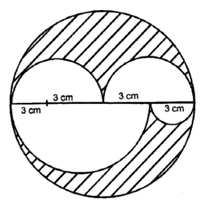1) C

2) C

3) B

4) A

5) B

6) D

7) C

8) C

9) A

10) D

11) D

12) B

13) B

14) A

15) A

16) C

17) C

18) A

19) A

20) C